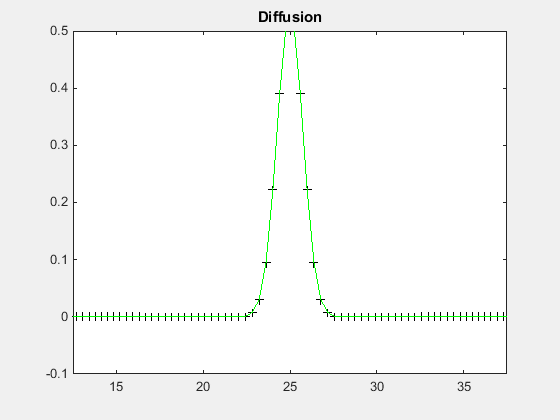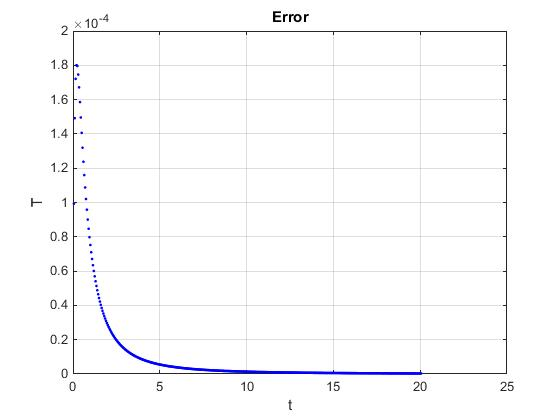# Presentation

Here the code for the diffusion equation. We took the Vibrating String code and modified it to show the diffusion phenomenon. The equation used is the well-known diffusion equation also the Heat Equation without source : \displaystyle f_t=\mu f_{xx} The resolution is the same that the vibrating string, a march in time for the time derivative and a differentiation matrix for the spatial derivative.

clear all; close all;

% parameters
N=200; % number of gridpoints
L=50; % domain length
mu=0.5; % thermic conductivity
dt=0.05; % time step
tmax=20; % final time
x0=L/2;
t0=0.5;

% differentiation matrices
scale=-2/L;
[x,DM] = chebdif(N,2);
dx=DM(:,:,1)*scale;
dxx=DM(:,:,2)*scale^2;
x=(x-1)/scale;
I=eye(N);

% system matrices
E=I;
A=mu*dxx;


# Boundary Conditions

We know that at the boundary conditions, the solution is zero, thus we put this value in the differentiation matrix like this :

% boundary conditions
E([1 N],:)=0;
A([1 N],:)=I([1 N],:);

% march in time matrix
M=(E-A*dt/2)\(E+A*dt/2);


# Initial Condition

For the initial condition we use the Gaussian function that represent well the diffusion at the initial time : \displaystyle T=\frac{1}{\sqrt{2\pi t_0}}exp\left(\frac{-(x-x_0)^2}{2t_0}\right)

% initial condition
q=1/sqrt(2*pi*t0)*exp(-(x-x0).^2/2/t0);

% marching loop
tvec=0:dt:tmax;
Nt=length(tvec);
filename = 'Diffusion.gif';
for ind=1:Nt
q=M*q; % one step forward
t=ind*dt;

Tt=1/sqrt(2*pi*(t+t0))*exp(-(x-x0).^2/2/(t+t0));

% plotting
subplot(1,2,1);
plot(x,q,'k+',x,Tt,'g-');
title('Diffusion');
xlabel('x'); ylabel('T');
axis([L/4,3*L/4,-0.1,0.5])

subplot(1,2,2);
plot(t,norm((q-Tt),2),'b.'); grid on; hold on
drawnow
title('Error')
xlabel('t'); ylabel('T');

end